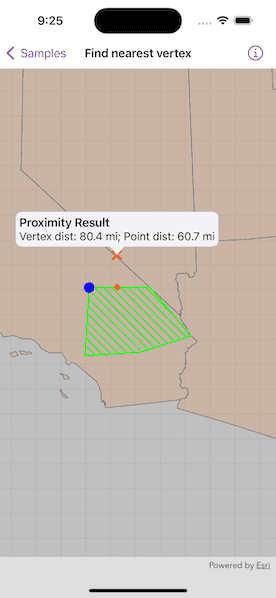# Find nearest vertex

Find the closest vertex and coordinate of a geometry to a point.## Use case

Determine the shortest distance between a location and the boundary of an area. For example, developers can snap imprecise user taps to a geometry if the tap is within a certain distance of the geometry.

## How to use the sample

Tap anywhere on the map. An orange cross will show at that location. A blue circle will show the polygon's nearest vertex to the point that was tapped. A red diamond will appear at the coordinate on the geometry that is nearest to the point that was tapped. If tapped inside the geometry, the red and orange markers will overlap. The information callout showing distance between the tapped point and the nearest vertex/coordinate will be updated with every new location tapped.

## How it works

1. Get a `Geometry` and a `Point` to check the nearest vertex against.
2. Call `class GeometryEngine.nearestVertex(in:to:)`.
3. Use the returned `ProximityResult` to get the `Point` representing the polygon vertex, and to determine the distance between that vertex and the tapped point.
4. Call `class GeometryEngine.nearestCoordinate(in:to:)`.
5. Use the returned `ProximityResult` to get the `Point` representing the coordinate on the polygon, and to determine the distance between that coordinate and the tapped point.

## Relevant API

• class GeometryEngine.nearestCoordinate(in:to:)
• class GeometryEngine.nearestVertex(in:to:)
• class GeometryEngine.normalizeCentralMeridian(of:)
• Geometry
• ProximityResult

The value of `ProximityResult.distance` is planar (Euclidean) distance. Planar distances are only accurate for geometries that have a defined projected coordinate system, which maintain the desired level of accuracy. The example polygon in this sample is defined in California State Plane Coordinate System - Zone 5 (WKID 2229), which maintains accuracy near Southern California. Accuracy declines outside the state plane zone.

## Tags

analysis, coordinate, geometry, nearest, proximity, vertex

## Sample Code

FindNearestVertexView.swift
Use dark colors for code blocksCopy
``````// Copyright 2023 Esri
//
// you may not use this file except in compliance with the License.
// You may obtain a copy of the License at
//
//
// Unless required by applicable law or agreed to in writing, software
// WITHOUT WARRANTIES OR CONDITIONS OF ANY KIND, either express or implied.
// See the License for the specific language governing permissions and

import ArcGIS
import SwiftUI

struct FindNearestVertexView: View {
/// The view model for the sample.
@StateObject private var model = Model()

/// A location callout placement.
@State var calloutPlacement: CalloutPlacement?

/// The tap location.
@State var tapLocation: Point!

var body: some View {
MapView(map: model.map, graphicsOverlays: [model.graphicsOverlay])
.onSingleTapGesture { _, mapPoint in
// Normalize map point.
guard let normalizedMapPoint = GeometryEngine.normalizeCentralMeridian(of: mapPoint) as? Point else { return }
tapLocation = normalizedMapPoint
if calloutPlacement == nil {
// Draw the point graphics.
model.updateNearestPoints(point: tapLocation)
// Show the callout at the tapped location.
calloutPlacement = CalloutPlacement.location(tapLocation)
} else {
// Remove points and hide callout.
model.tapLocationGraphic.geometry = nil
model.nearestCoordinateGraphic.geometry = nil
model.nearestVertexGraphic.geometry = nil
calloutPlacement = nil
}
}
.callout(placement: \$calloutPlacement.animation(.default.speed(2))) { _ in
Text("Proximity Result")
Text("Vertex dist: \(model.nearestVertexDistance); Point dist: \(model.nearestCoordinateDistance)")
.font(.callout)
}
}
}
}

private extension FindNearestVertexView {
/// The view model for the sample.
class Model: ObservableObject {
/// A map with a generalized US states feature layer and centered on
/// the example polygon in California.
let map: Map = {
let map = Map(spatialReference: .statePlaneCaliforniaZone5)

// Center map on the example polygon.
map.initialViewpoint = Viewpoint(
center: .sanBernardinoCounty.extent.center,
scale: 8e6
)

// Add US states feature layer to the map.
let usStatesGeneralizedLayer = FeatureLayer(
item: PortalItem(
portal: .arcGISOnline(connection: .anonymous),
id: .usStatesGeneralized
)
)

return map
}()

/// The graphics overlay for the point and polygon graphics.
let graphicsOverlay = GraphicsOverlay()

/// An orange cross graphic for the tap location point.
let tapLocationGraphic: Graphic = {
let symbol = SimpleMarkerSymbol(style: .x, color: .orange, size: 15)
return Graphic(symbol: symbol)
}()

/// A blue circle graphic for the nearest vertex point.
let nearestVertexGraphic: Graphic = {
let symbol = SimpleMarkerSymbol(style: .circle, color: .blue, size: 15)
return Graphic(symbol: symbol)
}()

/// A red diamond graphic for the nearest coordinate point.
let nearestCoordinateGraphic: Graphic = {
let symbol = SimpleMarkerSymbol(style: .diamond, color: .red, size: 10)
return Graphic(symbol: symbol)
}()

/// The nearest coordinate distance on the polygon to the tap location.
var nearestCoordinateDistance: String = ""

/// The nearest vertex distance on the polygon to the tap location.
var nearestVertexDistance: String = ""

init() {
// Create graphic for the example polygon.
let polygonFillSymbol = SimpleFillSymbol(
style: .forwardDiagonal,
color: .green,
outline: SimpleLineSymbol(style: .solid, color: .green, width: 2)
)
let polygonGraphic = Graphic(
geometry: .sanBernardinoCounty,
symbol: polygonFillSymbol
)

// Add graphics to the graphicOverlay.
polygonGraphic,
tapLocationGraphic,
nearestCoordinateGraphic,
nearestVertexGraphic
])
}

/// Draws the nearest coordinate and vertex to the point on the example polygon.
/// - Parameter point: A `Point` to measure against.
func updateNearestPoints(point: Point) {
// Get nearest vertex and coordinate to the point.
let nearestVertexResult = GeometryEngine.nearestVertex(in: .sanBernardinoCounty, to: point)!
let nearestCoordinateResult = GeometryEngine.nearestCoordinate(in: .sanBernardinoCounty, to: point)!

// Set the geometries for the tapped, nearest coordinate, and
// nearest vertex point graphics.
tapLocationGraphic.geometry = point
nearestVertexGraphic.geometry = nearestVertexResult.coordinate
nearestCoordinateGraphic.geometry = nearestCoordinateResult.coordinate

// The format style with a decimal point.
let formatStyle = Measurement<UnitLength>.FormatStyle(
width: .abbreviated,
numberFormatStyle: .number.precision(.fractionLength(1))
)

// Set the distance to the nearest vertex in the polygon.
let vertexDistance = Measurement(
value: nearestVertexResult.distance,
unit: UnitLength.feet
)
nearestVertexDistance = vertexDistance.formatted(formatStyle)

// Set the distance to the nearest coordinate in the polygon.
let coordinateDistance = Measurement(
value: nearestCoordinateResult.distance,
unit: UnitLength.feet
)
nearestCoordinateDistance = coordinateDistance.formatted(formatStyle)
}
}
}

private extension Geometry {
/// A polygon near San Bernardino County, California.
static let sanBernardinoCounty: ArcGIS.Polygon = {
let polygonBuilder = PolygonBuilder(spatialReference: .statePlaneCaliforniaZone5)
return polygonBuilder.toGeometry()
}()
}

private extension SpatialReference {
/// The spatial reference for the sample.
static var statePlaneCaliforniaZone5: Self { SpatialReference(wkid: WKID(2229)!)! }
}

private extension PortalItem.ID {
/// The ID used in the "US States Generalized" portal item.
static var usStatesGeneralized: Self { Self("8c2d6d7df8fa4142b0a1211c8dd66903")! }
}``````